## Friday, June 22, 2012

### The Fifth Degree: Part IV

I've been reading over what I posted yesterday about the cubic, where I identified a certain combination of the roots alpha, beta and gamma as the square root of a rational number: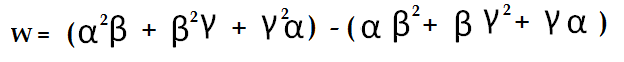Now I'm not all that happy with the way I explained this. All those terms in alpha-squared-beta, etc....those six functions really define everything about the structure of the algebraic field formed by adjoining alpha, beta, and gamma to the rationals. The field structure derives from the indistinguishability of the three roots. With respect to the rational numbers, the three roots are in general indistinguishable with respect to re-shuffling. This means that if two people evaluate any expression in alpha, beta and gamma, it doesn't matter if they make different choices for which one is which...the result, expressed in terms of rational numbers, will be the same either way.

Actually, this isn't always true. It's clearly not true for a cubic which factors, where one of the roots is rational and the others aren't. Even some irreducible cubics have roots that cannot always be shuffled indiscriminately. (These are cases where the roots have a cyclic relationship.) But in the most general case, the three roots can be freely interchanged with each other.

Let's look at the implications of this for our six terms in alpha-squared-beta, etc. Any re-shuffling of alpha, beta and gamma will result in a re-shuffling of our alpha-squared betas etc. For example, if we swap beta and gamma, then alpha-squared-beta becomes alpha-squared-gamma. But there is one interesting function in our alpha-squared-betas that has an interesting property. Consider: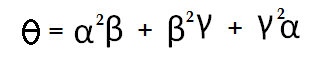If you start swapping alphas and betas with each other, you notice a peculiar thing. There are only two possible outcomes. Either you end up with theta unchanged, or you end up with what I'll call theta-prime: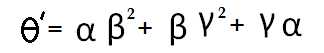Try it if you don't believe me. Any operation whereby you switch the alphas, betas and gammas with each other can only have one of two outcomes on the thetas: either they stay where they are, or they trade places with each other. And that means our thetas are the roots of a quadratic equation.

Furthermore, it's easy to see that they are the roots of a quadratic. Just take their sum and their product. Our thetas are not quite symmetric functions in alpha, beta and gamma. They are just a bit unsymmetric. But their sum and product are both symmetric functions, and as such, must have rational values. (As I pointed out last time, this property is inherited from the elementary symmetric functions in alpha, beta and gamma, which are just the coefficients of the cubic equation.)

When I tried to explain this yesterday, I basically started from the position that the difference of the thetas is the square root of a rational. Well, that's true for the roots of any quadratic equation, but I don't think it was the best way to make my point...not when I get around to generalizing the argument to equations of higher degree.

What do we really gain by the existence of the thetas? The huge consequence, the thing that Galois recognized, is this: that we have the field of rational numbers, and we have the field of algebraic numbers formed by adjoining the roots of the cubic to the rationals: these are the fields that we already knew about. But now we have a third field, intermediate between these two fields, which is formed by adjoining an ordinary square root to the rationals. And this intermediate field is the stepping stone which helps us reach our ultimate destination, the field which is known as the "splitting field" of the cubic.

Now, I took a course in this stuff many decades ago, and I distinctly remember that we supposedly reach the splitting field by adjoining nth roots of an element of each successive field. So having reached this intermediate field, you'd think we simply adjoin the cube root of some element and this generates the destination field.

Incredibly, it doesn't seem to work that way. We are, for the sake of argument, sitting in the field formed by adjoining the square root of seven to the rationals. Now take a typical element of this field, such as twelve take-away four root seven, and cube root it. Adjoin this number to the field we already had: this gives us: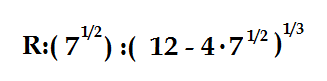where we've created this extension field first by adjoining the square root of an ordinary element of our starting field, the rationals; and then by adjoining the cube root of a typical element in the secondary field. And yet: this is not the splitting field of a cubic. We have to adjoin one more element: the conjugate of the last element we adjoined. It's not enough to tack on one without the other. We have to make both choices of the square root: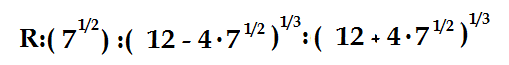And needless to say, it wasn't enough to adjoin just one of the cube roots: we have to adjoin all three cube roots of each of the two conjugates.

Is this new field the splitting field of our cubic? Actually, no. It's a bigger field than our splitting field. It includes elements that are not  part of the true splitting field, the field formed by the algebraic closure of alpha, beta, and gamma with the rationals. This field, the true splitting field, doesn't include our two conjugate cube root terms. Our alpha, beta and gamma are composed of the sums, suitably chosen, of those conjugate cube roots.

Furthermore, the whole ball of wax only works in the special circumstance that the product of our two conjugates happens to be a perfect cube. In the example I've chosen, we evaluate the product as 144 - 16*7 = 32....not a perfect cube. So this doesn't happen to be the splitting field of any cubic equation with rational coefficients.

It's all very peculiar.